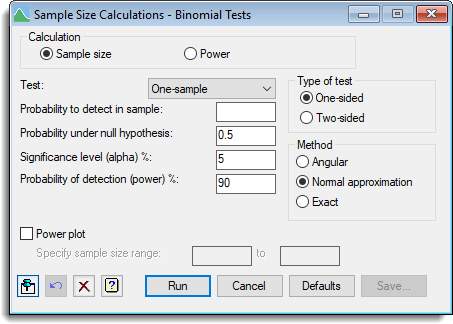1. Home
2. Sample Size Calculations – Binomial tests

# Sample Size Calculations – Binomial tests

Select menu: Stats | Sample Size | Binomial tests

Use this to calculate the number of replicates (sample size) or the power required for a binomial test. A one-sample binomial test assesses the evidence that the probability of success within a sample differs from some specific value. Alternatively, a two-sample test assess the evidence that probabilities within two samples are different.

Stats | Sample Size | Binomial Tests.
2. Fill in the fields as required then click Run.

After running the calculation you can save the results by clicking Save.## Calculation

Specifies the calculation to be carried out: Sample size, or Power.

## Test

Specifies the test to be carried out: One-sample, or Two-sample.

## Probability to detect in sample

For a one-sample test this specifies the probability that needs to be detected. Alternatively, for a two-sample test this specifies the anticipated probability within the first sample.

## Probability under null hypothesis

For a one-sample test this specifies the value from which the probability in the sample needs to be distinguished from (i.e. the value under the null hypothesis).

## Probability to detect in sample 2

For a two-sample test this specifies the probability within the second sample from which the probability in the first sample needs to be distinguished from.

## Significance level (alpha)%

Specifies the significance level at which the response is to be tested.

## Probability of detection (ower)%

For a sample size calculation this specifies the required probability for detection of the response (that is, the power of the test).

## Sample size

For a power calculation this specifies the sample size to be used to calculate the probability of detection (power).

## Replication ratio (sample 2: sample 1)

It is generally assumed that the sizes of the samples in the two-sample test should be equal. However, you can specify a ratio to indicate the size of the second sample in relation to the size of the first sample

## Power plot

When selected, produces a plot of power versus sample size. When Sample size is selected the plot is generated using 11 replication values centred around the required number of replicates for the requested power. When Power is selected you can specify a range of sample size values for the plot in the spaces provided.

## Type of test

Lets you choose either a One-sided or Two-sided test.

## Method

Specifies the type of binomial test that is to be done. The Normal approximation setting relates to a test based on the Normal approximation to the binomial distribution (see the BNTEST procedure), while the Angular setting is for a test using an angular transformation of the probabilities. The final setting, Exact, is available only for the one-sample test and assumes an exact test using the binomial distribution.

## Action IconsPin Controls whether to keep the dialog open when you click Run. When the pin is downthe dialog will remain open, otherwise when the pin is upthe dialog will close.Restore Restore names into edit fields and default settings.Clear Clear all fields and list boxes.Help Open the Help topic for this dialog.